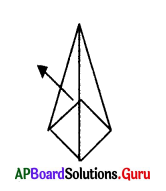Practice the AP 8th Class Maths Bits with Answers Chapter 13 Visualizing 3-D in 2-D on a regular basis so that you can attempt exams with utmost confidence.

## AP State Syllabus 8th Class Maths Bits 13th Lesson Visualizing 3-D in 2-D with Answers

Question 1.
Which of the following is a 3 – D figure?
A) Square
B) Rectangle
C) Cone
D) Triangle
C) Cone

Question 2.
Which of the following is a 2 -D figure?
A) Cube
B) Cuboid
C) Cylinder
D) Rectangle
D) RectangleQuestion 3.
No. of cubes of the given figure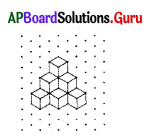A) 16
B) 14
C) 12
D) 10
D) 10

Question 4.
No. of faces of a square pyramid ……………
A) 4
B) 5
C) 3
D) 6
B) 5

Question 5.
A polyhedron has …………. minimum no. of faces.
A) 1
B) 2
C) 3
D) 4
D) 4

Question 6.
No. of edges of a cuboid are
A) 6
B) 10
C) 8
D) 12
D) 12

Question 7.
No. of vertices of a cube are
A) 4
B) 8
C) 6
D) 12
B) 8

Question 8.
No. of faces of a triangular pyramid are
A) 4
B) 6
C) 8
D) 2
A) 4

Question 9.
A hexagonal pyramid has …………… faces.
A) 4
B) 5
C) 6
D) 8
C) 6Question 10.
Which of the following is an Euler’s law ?
A) F + V = E + 2
B) F + V = E – 2
C) F – E = V – 2
D) F + E = V – 2
A) F + V = E + 2

Question 11.
The shape of a prism depend upon its ……………..
A) Base
B) Cone
C) Vertex
D) Number
A) Base

Question 12.
No. of faces of a pentagonal pyramid are ……………..
A) 6
B) 5
C) 4
D) 9
B) 5

Question 13.
The net of a cube is …………….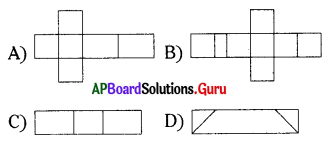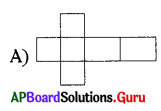Question 14.
The net for a cylinder is ……………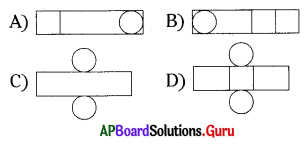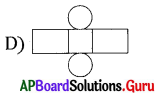Question 15.
No. of edges of a figure ……………..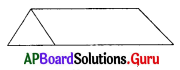A) 6
B) 10
C) 12
D) 9
D) 9

Question 16.
The Euler’s relation among no. of faces, vertices and edges is ……………
A) E + V = E
B) F + V = E + 2
C) F – V = E
D) F – V = E – 2
B) F + V = E + 2Question 17.
If the sides of the base of a regular pyramid are increases infinitely then the shape of its base is ……………….
A) Cylinder
B) Cone
C) Prism
D) None
B) Cone

Question 18.
Identify cuboid among the following.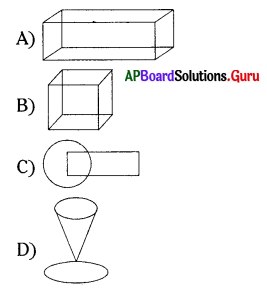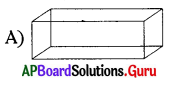Question 19.
Identify 3-D object from the following.
A) Sphere
B) Circle
C) Paper
D) None
A) Sphere

Question 20.
A polygon with 4 sides is called ………………
A) Triangle
C) Pentagon
D) Sexagon

Question 21.
Number of edges of a cuboid is …………………
A) 11
B) 10
C) 6
D) None
D) None

Question 22.
The name of given figure is ………………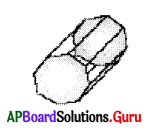A) Triangular prism
B) Octagonal prism
C) Pentagonal prism
B) Octagonal prism

Question 23.
The given figure indicates ……………..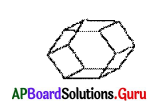A) Octagonal prism
B) Cylinder
C) Pentagonal prism
D) Triangular prism
A) Octagonal prismQuestion 24.
In the figure F = …………….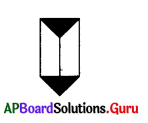A) 6
B) 3
C) 5
D) 9
C) 5

Question 25.
In the figure V = ………….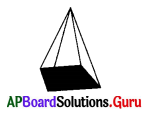A) 5
B) 6
C) 10
D) 4
A) 5

Question 26.
An object with more number of faces is ……………..
A) Circle
B) Paper
C) Square
D) Sphere
D) Sphere

Question 27.
How many cubes are there in the figure of 1 unit measure?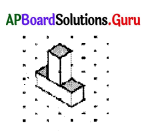A) 6
B) 4
C) 12
D) 5
D) 5

Question 28.
How many cubes are there in the figure of 1 unit measure?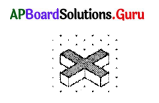A) 9
B) 14
C) 6
D) 2
A) 9

Question 29.
How many cubes are there in the figure of 1 unit measure?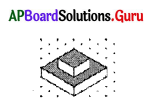A) 10
B) 20
C) 14
D) 13
B) 20

Question 30.
How many cubes are there in the figure of 1 unit measure ?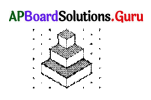A) 11
B) 13
C) 14
D) 16
C) 14

Question 31.
‘die’ is an example of ……………..
A) Triangle
B) Cube
C) Sphere
D) Pyramid
B) CubeQuestion 32.
Plasma T.V. is an example of …………….
A) Sphere
B) Rectangle
C) Triangle
D) Cuboid
D) Cuboid

Question 33.
Kite is an example of …………. object.
A) Two dimensional
B) 3D
C) Prism
D) Sphere
A) Two dimensional

Question 34.
In a cube f + v = …………….
A) 14
B) 16
C) 19
D) 20
A) 14

Question 35.
In a Pentagonal Pyramid E + 2 = ………………
A) 6
B) 9
C) 18
D) 12
D) 12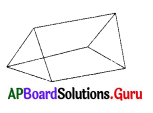Question 36.
No. of surfaces is …………….
A) 5
B) 6
C) 9
D) 10
A) 5

Question 37.
No. of edges is ………….
A) 12
B) 11
C) 9
D) 16
C) 9Question 38.
No. of vertices is ……………
A) 9
B) 6
C) 12
D) 13
B) 6

Question 39.
Number of edges of a cylinder is ……………….
A) 11
B) 10
C) 20
D) None
What does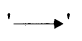in the figure indicate?